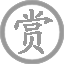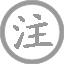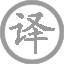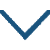和贾至舍人早朝大明宫之作

• jiǎ
• zhì
• shè
• rén
• zǎo
• cháo
• mínɡ
• ɡōnɡ
• zhī
• zuò
•
• tánɡ
•
• cén
• shēn
• mínɡ
• shǔ
• ɡuānɡ
• hán
•  ,
• yīnɡ
• zhuàn
• huánɡ
• zhōu
• chūn
• lán
•
• jīn
• què
• xiǎo
• zhōnɡ
• kāi
• wàn
•  ,
• jiē
• xiān
• zhànɡ
• yōnɡ
• qiān
• ɡuān
•
• huā
• yínɡ
• jiàn
• pèi
• xīnɡ
• chū
• luò
•
• liǔ
• jīng
• wèi
• gàn
•
• yǒu
• fènɡ
• huánɡ
• chí
• shànɡ
•  ,
• yánɡ
• chūn
• jiē
• nán
•

雄鸡啼鸣京城曙色光寒，
莺啼婉转皇宫春色正阑。
宫殿报晓钟声催开万户，
玉石台阶仪仗拥簇千官。
花迎朝臣佩剑繁星初落，
柳拂旌旗仙人盛零未干。
只有凤凰池上舍人诸客，
阳春高雅一曲和歌皆难。

注释

和（hè）：即和诗，是用来和答他人诗作的诗，依照别人诗词的格律或内容作诗词。可和韵，可不和韵。中书舍人：官名，时贾至任此职。大明宫：宫殿名，在长安禁苑南。
紫陌：指京师的街道。曙（shǔ）光：破晓时的阳光。
啭（zhuàn）：婉转的叫声。皇州：京都。阑：尽。
金阙（què）：皇宫金殿。万户：指皇宫中宫门。
玉阶：指皇宫中大明宫的台阶。仙仗：天子的仪仗。
剑佩：带剑、垂佩绶，都为高官之饰物，此指禁卫军的武装。
旌（jīng）旗：旗帜的总称。
凤凰池上客：指贾至。凤凰池，也称凤池，这里指中书省。
阳春：古曲名，即宋玉《对楚王问》中提到的《阳春》《白雪》，“国中属而和者不过数十人”，后以之比喻作品高妙而懂得的人很少。

赏析

杜甫、王维、岑参三首和《早朝大明宫》，其艺术成就都超过了贾至的原作。在诸和诗之中，杜甫的和诗以其格律谨严而著称，王维的和诗以其气象阔大而驰名，至于岑参的这首和诗，则以其押韵奇险、属对精工与用语之典丽而深获历代论者之盛誉。
诗一开头，先由作者在早、朝途中的所见所闻写起。这两句是说，诗人早朝上路之时，听见雄鸡正在报晓，看到东方刚微露曙光，觉得天气仍有些凉意；时值暮春三月，流莺百啭，在这京都之内不时可闻其鸣。首句写“鸡鸣”、“曙光”，交待早朝上路的时间，点题巧妙而又自然。在时间概念上也比贾至原诗首句“银烛朝天紫陌长”的笼统交待显得确切。次句写“莺啭”、“春色”，描绘京城暮春时节清晨的景色，呼应贾至原诗第二句“禁城春色晓苍苍”的写景。两相比较，贾诗所写之春景比较模糊，形象不鲜明，“春色晓苍苍”，艺术感染力实际上并很不强。究其原因，即在于拂晓之时天色尚暗，描写此时景物仅仅诉诸于视觉印象，其难度较大。岑参深谙其中奥秘，故其诗首联写景时，既写其所见之“曙光”、“春色”，又写其所闻之“鸡鸣”、“莺啭”，甚至写到其身心所感觉之“寒”，准确地抓住了暮春时节清晨之时景物和气候的特点，从视觉、听觉、感觉等不同角度进行描写，艺术感染力自然就强了不少。
“金阙晓钟开万户，玉阶仙仗拥千官”，与王维和诗的颔联一样，岑诗颔联联写的也是早朝时的场面：伴随着金銮殿里传出的朝钟声，一扇扇宫门依次而开；在汉自玉台阶两侧排列着皇家的仪仗，文武百官们按部而朝见皇帝。“金阙”、“玉阶”，其辞藻富丽堂皇，正适合表现皇宫的金璧辉煌和雕栏玉砌。以“金”对“玉”，以“万”，对“千”，其对仗典雅精工，又与早朝时庄严整肃之朝仪相谐。因此若论气象之阔大，岑诗此联诚逊于王诗，若沦辞藻之富丽与对仗之精工，则岑诗又在王诗之上。
岑诗的颈联颇得后世论者之青睐，南宋著名诗人杨万里在其著《诚斋诗话》中论及贾至等人的这组早朝大明宫唱和诗时曾说：“和此诗者，岑诗云‘花迎剑佩星初落，柳拂旌旗露未干’，最佳。”在杜甫、王维均参与唱和的情况下，岑诗此联能获得如此高的评价，是不容易的。此联的佳处即在于它既写了早朝的场面又抓住了时间早这一特点，既呼应贾至的原作又表现出岑诗的语言特色：鲜花迎接饰有佩剑的官员，柳条轻拂仪仗的旗帜，此时晓星方落，露犹未干。作为一首早朝诗，应处理好“早”与“朝”之关系，即在空问上应围绕朝见之场面来写，在时间上又要体现出一个“早”字。贾、杜、工三人的唱和诗，均以首联写早朝之时间，以中二联写一早朝之场面，唯岑诗此联能将“花迎剑佩”、“柳拂族旗”之场面与“星初落”、“露未干”之时辰融为一体，以一联表现了他人需两联才能交待清楚的内容。作为一首和诗，岑诗此联的景物描写又与贾至原诗互为呼应，进一步充实了对春色的描写。不过贾至的原作中，写景与朝见结合得不紧密，结果出现了首二联写景，颈联写朝见，尾联倡和的格局，对于一首早朝诗来说，其中心不突出，显得本末倒置。因此，王维在和其诗时，以中二联写朝见场面，突出了重点。但王诗也有不足，即忽视了贾至原作中的春色描写，与之呼应不紧。观王诗，竟通篇于春色未着一字，反倒用了不少笔墨不厌其详地一再写君臣之服饰，首联已云“翠云裘”，颈联又写“衮龙”，颔联则先写“衣冠”，又继以“冕旒”，语意颇嫌重复，而不腾出笔墨来呼应一下贾诗中之春色。前人对此有“衣冠冕旒，句中字面复见”之讥（《仇兆鳌《杜诗详注》卷五）。虽说王诗成就很高，此等不足毕竟是瑕不掩瑜，但相比之下，总是不如岑诗之唱和得体、一呼应紧密而又能避免其中心不突出之缺陷。最后，此联之语言亦颇能体现岑诗之特色。纵观贾、杜、王三诗之颈联，均有御炉香烟等字样，可见在早朝诗中写香烟之类已成司空见惯之例。岑参显然不满足于此等俗套，故全诗无一语道及御炉香烟而于此联写出了“剑”、“旗”、“星”、“露”等较之其他三作显得很新奇的景语。所谓边塞诗人与宫廷诗人之别，于此大概亦可略见一斑。
“独有凤池上客，阳春一曲和皆难。”诗的结尾，响应贾至的倡议：唯有身居凤池的中书省贾舍人，你这一曲高雅的阳春自雪和起来实在困难。说和诗难，倒不仅仅是出于对贾至的恭维，或是表现自己的谦逊，这“难”字确实流露出岑参内心的真实感受。一同和诗的王维、杜甫，都是久负盛名的大诗人，与之同和一诗确实不易。王维久任朝官，写起宫廷唱和诗来简直是轻车熟路；杜甫做诗刻苦，语不惊人死不休，又尤长于律诗。诗友在某种意义上又是诗敌，同和一诗实寓有一较短长之意，因此在这场高水平的竞赛中若无高超的手段是根本不可能争先的。后人在评价这组唱和诗时多以岑参此诗为最佳，其致胜之诀不外乎下列三点：
其一曰“押韵奇险”。写宫廷诗，古人多以富丽之辞藻充做韵脚，如贾诗之“御炉香”、“侍君王”、杜诗之“醉仙桃”、“有凤毛”、王诗之“拜冕旒”、“衰龙浮”等，均是其例。岑参不排斥富丽之辞藻，但更偏爱奇丽之辞藻，体现在此诗之韵脚上，便是“曙光寒”、“春色阑”、“和皆难”、“露未干”等出乎人们意料的辞藻与奇特的押韵。在宫廷诗中，“寒”、“阑”、“干’、“难”等韵脚是不大有人问津的，更不用说以之作为早朝诗的韵脚了，这些词汇，通常是用以表现衰残之景的，将它们写入早朝诗中而不减损富丽堂皇之气，需要有高超的写作技巧。稍一不慎，便成败笔，在前人看来这简直是在走独木桥，故称这些韵脚为险韵。正是在这种他人通常不敢尝试之处，岑参成功地进行了尝试，收到了后世公认的奇特的艺术效果。具体说来，“寒”通常会引起人们的蜷曲畏缩之感，但岑诗的“鸡鸣紫陌曙光寒”给人的感觉便全然不同，雄鸡一唱天下白，黑暗即将让位于光明，那鸡鸣令人振奋，那曙光令人憧憬，那暮春清晨的微寒令人惬意。奇妙的艺术效果的取得就在于诗人在以“寒”为韵脚时恰当地进行了搭配，这一点在“阑”、“干”、“难”等韵脚上同样得到了体现。“阑”本用于几写残景，但诗人配之以“春色”、配之以“莺啭”，效果便截然相反。春阑不同于秋阑，花虽疏而叶更茂，红虽瘦而绿益肥，加之以流莺百咐，越发显得生机勃勃。其他如，’干”、“难”等韵脚，均各有妙用。在美学领域中，也处处存在着辨证法。岑诗中这几个韵脚，押得虽险而丽，虽丽又奇，颇能体现岑诗尚奇丽之特点。
其二曰“对仗精工”。对于律诗来讲，中二联对仗即已足矣，岑诗多用一联对仗，意在与早朝时那种左右分班、文武对列的朝仪相协调一致，以诗歌形式上的工整表现诗歌内容即早朝场面的严整。另外前人已经指出，此诗首联以“紫”对“皇”，极为典丽，岑参此诗对仗之精于此亦可见一斑。在这四首唱和诗中，论对仗之精工典丽，唯杜诗可与岑参此诗相敌。
其三曰“辞藻典丽堂皇”。岑参虽尚奇丽，但并不排斥典丽堂皇之语。在岑参此诗中，虽然有若于奇险之韵脚，但也有不少典丽堂皇之造语，其例如“紫陌”、“皇州”、“金阙”、“玉阶”等，绝不亚于其他三诗。早朝诗毕竟要写宫廷气象，若一味追求奇险就有可能破坏诗中画面的和谐。岑诗虽押险韵而未过份，又用了若干典丽堂皇之辞藻表现宫廷气象，恰到好处地取得了平衡。其诗虽奇而又未离格，达到了奇不离正，正中有奇，得心应手的境界。• chánɡ
• hèn
• ɡē
•
• tánɡ
•
• bái
• hàn
• huánɡ
• zhònɡ
• qīnɡ
• ɡuó
•  ,
• duō
• nián
• qiú
•
• yánɡ
• jiā
• yǒu
• chū
• zhǎnɡ
• chénɡ
•  ,
• yǎnɡ
• zài
• shēn
• ɡuī
• rén
• wèi
• shí
•
• tiān
• shēnɡ
• zhì
• nán
•  ,
• zhāo
• xuǎn
• zài
• jūn
• wánɡ
•
• huí
• móu
• xiào
• bǎi
• mèi
• shēnɡ
•  ,
• liù
• ɡōnɡ
• fěn
• dài
• yán
•
• chūn
• hán
• huá
• qīnɡ
• chí
•  ,
• wēn
• quán
• shuǐ
• huá
• nínɡ
• zhī
•
• shì
• ér
• jiāo
•  ,
• shǐ
• shì
• xīn
• chénɡ
• ēn
• shí
•
• yún
• bìn
• huā
• yán
• jīn
• yáo
•  ,
• rónɡ
• zhànɡ
• nuǎn
• chūn
• xiāo
•
• chūn
• xiāo
• duǎn
• ɡāo
•  ,
• cónɡ
• jūn
• wánɡ
• zǎo
• cháo
•
• chénɡ
• huān
• shì
• yàn
• xián
• xiá
•  ,
• chūn
• cónɡ
• chūn
• yóu
• zhuān
•
• hòu
• ɡōnɡ
• jiā
• sān
• qiān
• rén
•  ,
• sān
• qiān
• chǒnɡ
• ài
• zài
• shēn
•
• jīn
• zhuānɡ
• chénɡ
• jiāo
• shì
•  ,
• lóu
• yàn
• zuì
• chūn
•
• mèi
• xiōnɡ
• jiē
• liè
•  ,
• lián
• ɡuānɡ
• cǎi
• shēnɡ
• mén
•
• suí
• lìnɡ
• tiān
• xià
• xīn
•  ,
• zhònɡ
• shēnɡ
• nán
• zhònɡ
• shēnɡ
•
• ɡōnɡ
• ɡāo
• chù
• qīnɡ
• yún
•  ,
• xiān
• yuè
• fēng
• piāo
• chù
• chù
• wén
•
• huǎn
• ɡē
• màn
• nínɡ
• zhú
•  ,
• jìn
• jūn
• wánɡ
• kàn
•
• yánɡ
• ɡǔ
• dònɡ
• lái
•  ,
• jīnɡ
• chánɡ
•
• jiǔ
• chóng
• chénɡ
• què
• yān
• chén
• shēnɡ
•  ,
• qiān
• shèng
• wàn
• 西
• nán
• xínɡ
•
• cuì
• huá
• yáo
• yáo
• xínɡ
• zhǐ
•  ,
• 西
• chū
• mén
• bǎi
•
• liù
• jūn
• nài
•  ,
• wǎn
• zhuǎn
• é
• méi
• qián
•
• huā
• diàn
• wěi
• rén
• shōu
•  ,
• cuì
• qiáo
• jīn
• què
• sāo
• tóu
•
• jūn
• wánɡ
• yǎn
• miàn
• jiù
•  ,
• huí
• kàn
• xuè
• lèi
• xiānɡ
• huò
• liú
•
• huánɡ
• āi
• sǎn
• màn
• fēnɡ
• xiāo
• suǒ
•  ,
• yún
• zhàn
• yínɡ
• dēnɡ
• jiàn
• ɡé
•
• é
• méi
• shān
• xià
• shǎo
• rén
• xínɡ
•  ,
• jīnɡ
• ɡuānɡ
•
• shǔ
• jiānɡ
• shuǐ
• shǔ
• shān
• qīnɡ
•  ,
• shènɡ
• zhǔ
• zhāo
• zhāo
• qínɡ
•
• xínɡ
• ɡōnɡ
• jiàn
• yuè
• shānɡ
• xīn
•  ,
• wén
• línɡ
• duàn
• chánɡ
• shēnɡ
•
• tiān
• xuán
• zhuǎn
• huí
• lónɡ
•  ,
• dào
• chóu
• chú
• nénɡ
•
• wéi
• xià
• zhōnɡ
•  ,
• jiàn
• yán
• kōnɡ
• chù
•
• jūn
• chén
• xiānɡ
• ɡù
• jìn
• zhān
•  ,
• dōnɡ
• wànɡ
• mén
• xìn
• ɡuī
•
• ɡuī
• lái
• chí
• yuàn
• jiē
• jiù
•  ,
• tài
• rónɡ
• wèi
• yānɡ
• liǔ
•
• rónɡ
• miàn
• liǔ
• méi
•  ,
• duì
• lèi
• chuí
•
• chūn
• fēnɡ
• táo
• huā
• kāi
•  ,
• qiū
• tónɡ
• luò
• shí
•
• 西
• ɡōnɡ
• nán
• nèi
• duō
• qiū
• cǎo
•  ,
• luò
• mǎn
• jiē
• hónɡ
• sǎo
•
• yuán
• bái
• xīn
•  ,
• jiāo
• fánɡ
• ē
• jiàn
• qīnɡ
• é
• lǎo
•
• diàn殿
• yínɡ
• fēi
• qiǎo
• rán
•  ,
• ɡū
• dēnɡ
• tiǎo
• jìn
• wèi
• chénɡ
• mián
•
• chí
• chí
• zhōnɡ
• ɡǔ
• chū
• chánɡ
•  ,
• ɡěnɡ
• ɡěnɡ
• xīnɡ
• shǔ
• tiān
•
• yuān
• yānɡ
• lěnɡ
• shuānɡ
• huá
• zhònɡ
•  ,
• fěi
• cuì
• qīn
• hán
• shuí
• ɡònɡ
•
• yōu
• yōu
• shēnɡ
• bié
• jīnɡ
• nián
•  ,
• hún
• cénɡ
• lái
• mènɡ
•
• lín
• qiónɡ
• dào
• shì
• hónɡ鸿
•  ,
• nénɡ
• jīnɡ
• chénɡ
• zhì
• hún
•
• wèi
• ɡǎn
• jūn
• wánɡ
• zhǎn
• zhuǎn
•  ,
• suí
• jiào
• fānɡ
• shì
• yīn
• qín
•
• pái
• kōnɡ
• bēn
• diàn
•  ,
• shēnɡ
• tiān
• qiú
• zhī
• biàn
•
• shànɡ
• qiónɡ
• luò
• xià
• huánɡ
• quán
•  ,
• liǎnɡ
• chù
• mánɡ
• mánɡ
• jiē
• jiàn
•
• wén
• hǎi
• shànɡ
• yǒu
• xiān
• shān
•  ,
• shān
• zài
• piāo
• miǎo
• jiān
•
• lóu
• ɡé
• línɡ
• lónɡ
• yún
•  ,
• zhōnɡ
• chuò
• yuē
• duō
• xiān
•
• zhōnɡ
• yǒu
• rén
• tài
• zhēn
•  ,
• xuě
• huā
• mào
• cēn
• shì
•
• jīn
• quē
• 西
• xiānɡ
• kòu
• jiōnɡ
•  ,
• zhuǎn
• jiào
• xiǎo
• bào
• shuānɡ
• chénɡ
•
• wén
• dào
• hàn
• jiā
• tiān
• shǐ使
•  ,
• jiǔ
• huá
• zhànɡ
• mènɡ
• hún
• jīnɡ
•
• lǎn
• tuī
• zhěn
• pái
• huái
•  ,
• zhū
• yín
• pínɡ
• kāi
•
• yún
• bàn
• piān
• xīn
• shuì
• jué
•  ,
• huā
• ɡuān
• zhěnɡ
• xià
• tánɡ
• lái
•
• fēnɡ
• chuī
• xiān
• mèi
• piāo
• yáo
•  ,
• yóu
• chánɡ
•
• rónɡ
• lèi
• lán
• ɡān
•  ,
• huā
• zhī
• chūn
• dài
•
• hán
• qínɡ
• nínɡ
• xiè
• jūn
• wánɡ
•  ,
• bié
• yīn
• rónɡ
• liǎnɡ
• miǎo
• mánɡ
•
• zhāo
• yánɡ
• diàn殿
• ēn
• ài
• jué
•  ,
• pénɡ
• lái
• ɡōnɡ
• zhōnɡ
• yuè
• cháng
•
• huí
• tóu
• xià
• wànɡ
• rén
• huán
• chù
•  ,
• jiàn
• chánɡ
• ān
• jiàn
• chén
•
• wéi
• jiānɡ
• jiù
• biǎo
• shēn
• qínɡ
•  ,
• diàn
• jīn
• chāi
• jiānɡ
•
• chāi
• liú
• ɡǔ
• shàn
•  ,
• chāi
• huánɡ
• jīn
• fēn
• diàn
•
• dàn
• jiāo
• xīn
• jīn
• diàn
• jiān
•  ,
• tiān
• shànɡ
• rén
• jiān
• huì
• xiānɡ
• jiàn
•
• lín
• bié
• yīn
• qín
• zhònɡ
•  ,
• zhōnɡ
• yǒu
• shì
• liǎnɡ
• xīn
• zhī
•
• yuè
• chánɡ
• shēnɡ
• diàn殿
•  ,
• bàn
• rén
• shí
•
• zài
• tiān
• yuàn
• zuò
• niǎo
•  ,
• zài
• yuàn
• wéi
• lián
• zhī
•
• tiān
• chánɡ
• jiǔ
• yǒu
• shí
• jìn
•  ,
• hèn
• mián
• mián
• jué
•• xiàng
•
• táng
•
• liú
• zhū
• què
• qiáo
• biān
• cǎo
• huā
•
• xiàng
• kǒu
• yáng
• xiá
•
• jiù
• shí
• wáng
• xiè
• táng
• qián
• yàn
•
• fēi
• xún
• cháng
• bǎi
• xìng
• jiā
•• cháng
• gān
• xínɡ
•
• tánɡ
•
• cuī
• hào
• jūn
• jiā
• chù
• zhù
•  ,
• qiè
• zhù
• zài
• hénɡ
• tánɡ
•
• tínɡ
• chuán
• zàn
• jiè
• wèn
•  ,
• huò
• kǒnɡ
• shì
• tónɡ
• xiānɡ
••
• jiàn
• wài
• yòu
• yán
• bié
•
• tánɡ
•
• shí
• nián
• luàn
• hòu
•  ,
• zhǎnɡ
• xiānɡ
• fénɡ
•
• wèn
• xìnɡ
• jīnɡ
• chū
• jiàn
•  ,
• chēnɡ
• mínɡ
• jiù
• rónɡ
•
• bié
• lái
• cānɡ
• hǎi
• shì
•  ,
• tiān
• zhōnɡ
•
• mínɡ
• línɡ
• dào
•  ,
• qiū
• shān
• yòu
• chóng
•• xiānɡ
•
• tánɡ
•
• wánɡ
• wéi
• hónɡ
• dòu
• shēnɡ
• nán
• ɡuó
•  ,
• chūn
• lái
• zhī
•
• yuàn
• jūn
• duō
• cǎi
• xié
•  ,
• zuì
• xiānɡ
•微信公众号